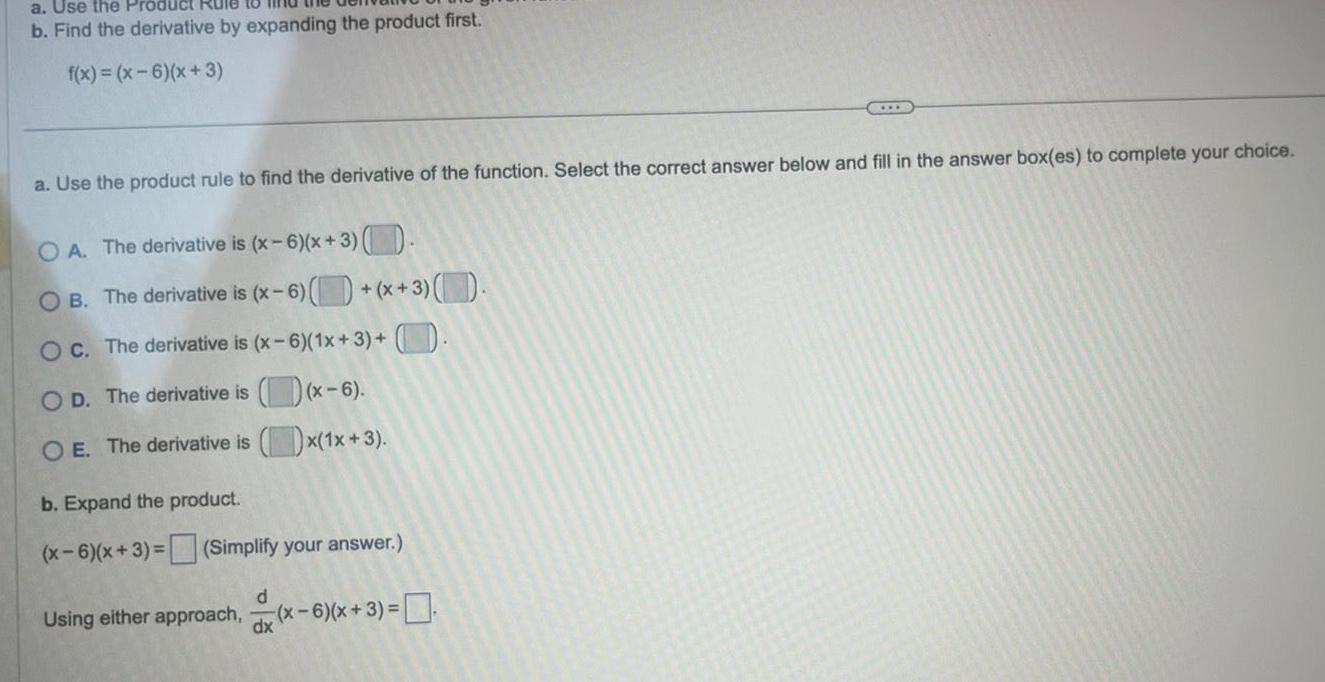Question:

# a Use the Product Rule to b Find the derivative by expanding

Last updated: 9/16/2023a Use the Product Rule to b Find the derivative by expanding the product first f x x 6 x 3 a Use the product rule to find the derivative of the function Select the correct answer below and fill in the answer box es to complete your choice O A The derivative is x 6 x 3 OB The derivative is x 6 x 3 O c The derivative is x 6 1x 3 OD The derivative is x 6 O E The derivative is x 1x 3 b Expand the product x 6 x 3 Simplify your answer Using either approach x 6 x 3 d dx